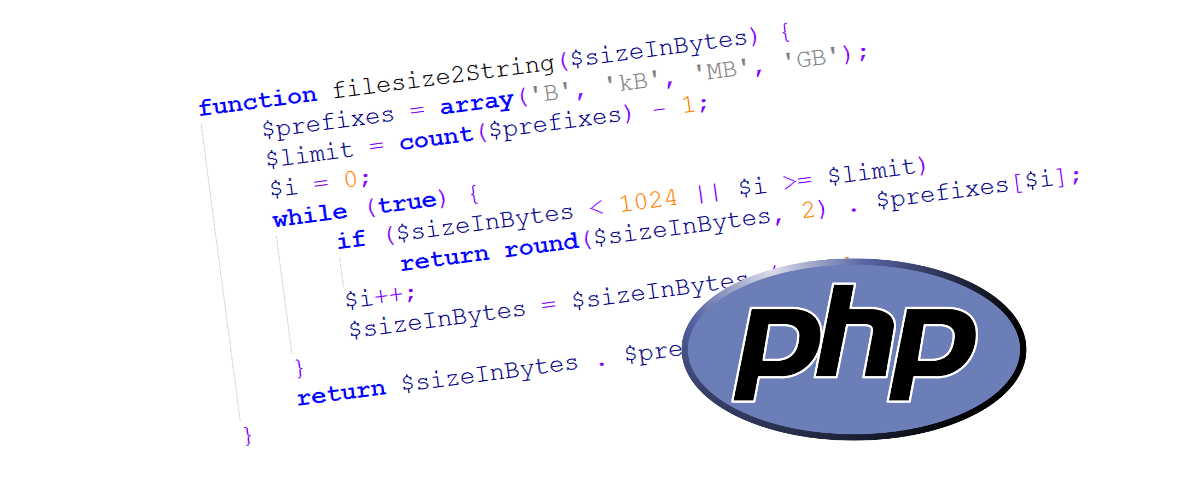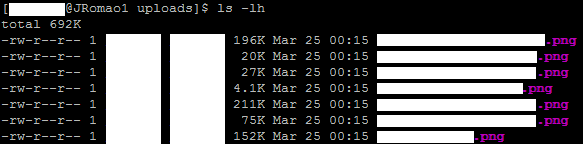If, like me, you have to create software that deals with files, it will probably come in handy to show your users how big the files are, so that they know what they’re getting into when they click the download button. And since not everybody has a computer background, looking at how many bytes a file has is not the best solution. That’s why we have units of measurement for files that are more human readable. A kilobyte has 1024 bytes, a megabyte has 1024 kilobytes and so on.

Unix systems do that when listing files by using the -h flag option:But other than that, we have to rely on the system functions that only return the size in bytes and work with that. This small but very useful function in PHP gets the size in bytes and by using a simple loop.
First, we define what the extensions for each measurement unit are, in a simple PHP array:

`\$prefixes = array('B', 'kB', 'MB', 'GB');`

Because we are only defining a limited set of units, we have to create a limit for how many loops the function can execute.  In this case, we know that there can only be 4 loops. The value of \$limit will be 3 (the size of \$prefixes minus 1) because PHP array indexes start at 0.

```\$limit = count(\$prefixes) - 1;
```

And now, since for every loop there is a corresponding unit of measurement, inside every loop we must check if this is the correct unit. How? By comparing the filesize to 1024 (2 to the power of 10). If the filesize is smaller than 1024 then the current unit of measurement is the right one to be used. For appearence purposes, we will also round the filesize to the second decimal and, finally, we append the unit to the calculated size.

```if (\$sizeInBytes < 1024 || \$i >= \$limit)
return round(\$sizeInBytes, 2) . \$prefixes[\$i];
```

If the filesize is still bigger than 1024, we just divide it by 1024 and go for the next loop.

```\$i++;
\$sizeInBytes = \$sizeInBytes / 1024;```

Full code below:

```function filesize2String(\$sizeInBytes) {
\$prefixes = array('B', 'kB', 'MB', 'GB');
\$limit = count(\$prefixes) - 1;
\$i = 0;
while (true) {
if (\$sizeInBytes < 1024 || \$i >= \$limit)
return round(\$sizeInBytes, 2) . \$prefixes[\$i];
\$i++;
\$sizeInBytes = \$sizeInBytes / 1024;
}
return \$sizeInBytes . \$prefixes[\$i];
}```